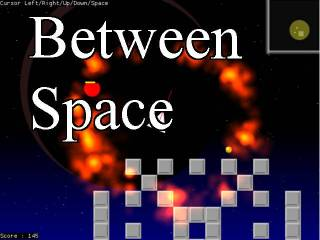-=+=- -=+=- -=+=- -=+=- -=+=- -=+=- -=+=- -=+=- -=+=- -=+=- -=+=- -=+=- -=+=- -=+=- -=+=- -=+=- -=+=- -=+=- -=+=- -=+=- -=+=- -=+=- -=+=- -=+=- -=+=- -=+=- -=+=- -=+=- -=+=- -=+=- (c) WidthPadding Industries 1987 0|426|0 -=+=- -=+=- -=+=- -=+=- -=+=- -=+=- -=+=- -=+=- -=+=- -=+=- -=+=- -=+=- -=+=- -=+=- -=+=- -=+=- -=+=- -=+=- -=+=- -=+=- -=+=- -=+=- -=+=- -=+=- -=+=- -=+=- -=+=- -=+=- -=+=- -=+=-
SoCoder -> Showcase Home -> Shoot 'em Up

PakzCreated : 18 December 2016
Edited : 18 December 2016
Language : Monkey

### Between Space

2d topdown space shooter with pickups and drones

Play and view code
ScreenshotsI have been working on this for a day or two or so. I think I am done with it for the time.

Link is to my blog post with the flash version playable there. Code is there to.

Here is the link to the html5 version :
https://cromdesi.home.xs4all.nl/html5/betweenspacegame/MonkeyGame.html

## Latest Update

Last detail was noising up the planet in the background. It still does not look really special though.

Added : 5 different Pickups, sideway shooting, backward shooting, regular shooting, artificial enemies(drones), and particle fire effect.